Note: This is a working paper which will be expanded/updated frequently. All suggestions for improvement are welcome. The directory deleeuwpdx.net/pubfolders/shepard has a pdf version, the complete Rmd file with all code chunks, the bib file, and the R source code.

# 1 Introduction

After Torgerson (1958) had more or less finalized the treatment of metric multidimensional scaling, Shepard had been searching for the function relating proximity judgments and distance, in what we now call the Shepard diagram (De Leeuw and Mair (2015)). His theory (Shepard (1957)) suggested a negative exponential relationship, but the experimental results were not convincing. The next major step was to let the data determine the nature of the relationship, and showing that this could be done by using only ordinal proximity information. This lead to the groundbreaking papers Shepard (1962a) and Shepard (1962b). Although Shepard’s contributions were certainly not forgotten, they were definitely overshadowed by the corresponding papers Kruskal (1964a) and Kruskal (1964b), published two years later by Shepard’s “co-worker” and “mathematical colleague” Joseph Kruskal. In Google Scholar the Shepard papers have 2364 and 1063 citations, the Kruskal papers have 6167 and 4167 (per 01/17/2017).

Shepard was well aware of the importance of his 1962 papers, which were the cumulation of a long list of his earlier contributions to mapping measures of proximity.

I have always regarded the development reported in this paper as one of my most original and significant accomplishments.

In the 1962 papers he developed an iterative technique, and a corresponding computer program, that maintains monotonicity between distances and dissimilarities while concentrating the variance of the configuration as much as possible in a small number of dimensions.

From Shepard (1979):

The idea of representing objects (such as colors, sounds, shapes, faces, word meanings, etc.) as points in space, in such a way that the distances between the points represented the perceived similarities between the objects, had occurred to me while I was an undergraduate student in 1951. But it was not until ten years later, when I gained access to the powerful computing facilities at the Bell Telephone Laboratories, that I conceived of an iterative process for reconstructing the implied spatial configuration even when the form of the monotone function relating similarity and distance was completely unknown.

After a period of trial-and-error adjustment of the parameters of the iterative process, success came with dramatic suddenness on March 17, 1961. According to the computer log, it was at precisely 2.33 p.m. EST on that day that the iterative process first converged to a stationary configuration, revealing a remarkably exact recovery of an underlying test configuration. The excitement of that moment was rivaled only by the birth of my daughter on the very next day. Since then my daughter has developed into a fine young woman; and, thanks in part to the subsequent contributions of my mathematical colleague Joseph Kruskal, nonmetric multidimensional scaling is now finding wide application throughout the cognitive, behavioral, and biomedical sciences.

Kruskal was politely critical of Shepard’s work. From Kruskal (1964a), page 2:

However, Shepard’s technique still lacks a solid logical foundation. Most notably, and in common with most other authors, he does not give a mathematically explicit definition of what constitutes a solution. He places the monotone relationship as the central feature, but points out (Shepard (1962a), p. 128) that a low-dimensional solution cannot be expected to satisfy this criterion perfectly. He introduces a measure of departure $$\delta$$ from this condition (Shepard 1962a, 136–37) but gives it only secondary importance as a criterion for deciding when to terminate his iterative process. His iterative process itself implies still another way of measuring the departure from monotonicity.

And again, on page 6-7, there is an implicit criticism of Shepard’s use of the original proximity measures to define fit.

Should we measure deviations between the curve and stars along the distance axis or along the dissimilarity axis? The answer is “along the distance axis.” For if we measure them along the dissimilarity axis, we shall find ourselves doing arithmetic with dissimilarities. This we must not do, because we are committed to using only the rank ordering of the dissimilarities.

From subsequent publications, and from some personal communications as well, I get the impression that Shepard interpreted Kruskal’s contribution as a relatively minor technical improvement of a procedure he had invented and published. I remember the consternation when one of the versions of the KYST program for MDS stated that KYST was short for Kruskal-Young-Seery-Torgerson. While Judith B. Seery, a programmer at Bell Labs, certainly worked on the program, later versions made the important correction that KYST stood for Kruskal-Young-Shepard-Torgerson. There seems to be some subtle acrimony in Shepard’s retelling of the history. Shepard (1974), p 376-377:

Although my original method generally yielded spatial configurations that appeared indistinguishable from those furnished by subsequent methods, my mathematical colleague Joseph Kruskal (Kruskal (1964a)) soon noted that the precise measure of departure from monotonicity that was being minimized by the method was neither explicitly defined nor even known to exist in an explicitly definable form. Thus, despite the intuitive plausibility and practical success of the method, it lacked the conceptual advantage of a strict mathematical specification of exactly what problem was being solved. Moreover, in the absence of an explicitly defined loss function, general techniques for the minimization of such functions (notably, gradient methods) were not apparently applicable. The iterative method that was used consequently appeared somewhat ad hoc.

Now however, some twelve years following my initial report of that method, my two former associates at the Bell Laboratories, Joe Kruskal (who succeeds me as President of this Society) and Doug Carroll (who has just been elected to succeed him), have jointly discovered that, unbeknown to all of us, my original method was in fact equivalent to a gradient method. Moreover they have even determined that the explicit form of the measure of departure from monotonicity that was (implicitly) minimized is one that appears to be quite reasonable (Kruskal and Carroll, personal communication; see Kruskal [in press]). Indeed, the method of optimization that was used apparently turns out, with a minor alteration in a normalizing factor, to belong to a class of “interchange methods” that Jan de Leeuw (personal communication) has recently shown to offer some advantages in the avoidance of merely local minima. However, these recently discovered aspects of my original method are mentioned, here, for their inherent or historical interest only; they do not form the basis for any of the recommendations that I shall offer in what follows.

I am not sure what I communicated in 1973 to Shepard. One of the purposes of this current paper is to try to reconstruct what I could possibly have meant by “interchange methods”. There is a major clue in the references of Kruskal (1977). Kruskal refers to a paper of mine from 1975, supposedly “in press”, titled “Nonmetric Scaling with Rearrangement Methods”. I vaguely remember an unpublished manuscript with a title like that, written in 1973-1974 while I was visiting Bell Labs. It’s irretrievably lost. We’ll just pretend it still exists (De Leeuw (1973a)). I will try to reconstruct some if it. The claim that Shepard’s original method was “equivalent to a gradient method” that minimized a well defined “measure of departure from monotonicity” still deserves some attention.

I agree that Kruskal’s improvement of Shepard’s technique was “mostly technical”, but it definitely was not “merely technical”. The use of monotone regression, the emphasis on explicit loss functions, and the use of gradient methods, created a whole new area of research in psychometrics and multivariate analysis. Meulman’s contribution on page 1194-1197 of Heiser et al. (2016) has some additional remarks on the impact of Kruskal’s work.

# 2 Shepard’s Method

A complete reconstruction of Shepard’s method would only be possible if we had the original FORTRAN program, but that has disappeared into the folds of time. We do have, however, Kruskal and Carroll (1977), which discusses the gradient interpretation and the rearrangement basis of the loss function.

• It was looking for a configuration in which distances were as monotonic as possible with the given measures of proximity.
• It was looking for a configuration which had as much variance as possible concentrated in the first few principal components.

In other words, it was a form of full-dimensional scaling in which configurations vary in $$n-1$$ dimensions. After convergence the first $$p$$ principal components of the result are reported as the $$p$$-dimensional solution.

Because of the two different objectives the displacement of points in an iteration was a compromise of two different displacements: one to improve monotonicity and one to improve low-dimensionality. In this paper we will only look at the deviations from monotonicity and, unlike Shepard, we will only look at configurations of a fixed dimension $$p$$. In this, of course, we follow Kruskal. Versions of Shepard’s full-dimensional method will be treated in another paper.

We change the notation somewhat, and we shift from working with measures of proximity to working with dissimilarities. To measure departure from monotonicity we compare a vector of dissimilarities $$\delta$$ and a vector of distances $$d(x)$$. Distances are defined as $$d_k(x)=\sqrt{x'A_kx}$$, where the $$A_k$$ are matrices of the form $$(e_i-e_j)(e_i-e_j)'$$, or direct sums of $$p$$ copies of such matrices (De Leeuw (1993)).

The loss function implicitly used by Shepard to measure departure from monotonicity is $\begin{equation}\label{E:loss1} \sigma^\star(x):=\sum_{k=1}^K(\hat\delta_k-\delta_k)d_k(x). \end{equation}$

Here the $$\hat\delta_k$$ are dissimilarities permuted in such a way that they are monotonic with the distances. Thus $$\hat{\delta}_k$$ is a function of both the numerical values of the dissimilarities and the rank order of the distances. We clearly perform “arithmetic with dissimilarities”, although I am not convinced that is necessarily a bad thing. Loss function $$\eqref{E:loss1}$$ does not occur in Shepard (1962a), but we infer it, basically by looking at his formula for displacements to optimize monotonicity. So far, we have not shown that $$\sigma_M$$ is continuous, let alone differentiable.

Using the classical rearrangement inequality (for example, Hardy, Littlewood, and Polya (1952), section 10.2, theorem 368) we can write $\begin{equation}\label{E:loss2} \sigma^\star(x)=\max_{\pi}\sum_{k=1}^K d_k(x)\delta_{\pi(k)}-\sum_{k=1}^K d_k(x)\delta_k, \end{equation}$ where $$\pi$$ varies over the set of permutations of $$\{1,\cdots,K\}$$. An alternative notation, which is sometimes useful, is $\begin{equation}\label{E:loss3} \sigma^\star(x)=\max_{P}\ \delta'Pd(x)-\delta'd(x), \end{equation}$

with $$P$$ varying over all permutation matrices. The representation using the rearrangement inequality shows that $$\sigma^\star$$ is non-negative, and $$\sigma^\star(x)=0$$ if and only if there are no violations of monotonicity. Thus it is a proper loss function for non-metric scaling. The representation $$\eqref{E:loss2}$$ shows that $$\sigma^\star$$ is the difference of two convex functions, which implies it is continuous and differentiable almost everywhere.

We assume throughout that $$d_k(x)>0$$ for all $$k$$. If there are no ties in $$\delta$$ or $$d(x)$$ then the maximizing permutation $$\hat\pi$$ is unique, and, by Danskin’s theorem (Danskin (1966)), $$\sigma_M$$ is differentiable with $\begin{equation}\label{E:dsm} \mathcal{D}\sigma^\star(x)=\sum_{k=1}^K\frac{\delta_{\hat\pi(k)}-\delta_k}{d_k(x)}A_kx \end{equation}$

Equation $$\eqref{E:dsm}$$ is basically the same as formula (4) on page 134 of Shepard (1962a).

Of course directly minimizing $$\sigma_M$$ over $$x$$ is not a useful technique, because if we set $$x=0$$ then we trivially find $$\sigma_M(x)=0$$. Thus we need some form of normalization. Shepard (1962a), page 135, says

Finally, at the end of each iteration the adjusted coordinates undergo a “similarity” transformation to re-center the centroid at the origin and to re-scale all distances so that the mean interpoint separation is maintained at unity. (p 135)

which seems to imply normalization by $$\sum_{k=1}^K d_k(x)=1$$. Thus we set $\begin{equation}\label{E:final} \sigma(x)=\frac{\sum_{k=1}^K(\hat\delta_k-\delta_k)d_k(x)}{\sum_{k=1}^Kd_k(x)} \end{equation}$ which makes $\begin{equation}\label{E:grad} \mathcal{D}\sigma(x)=\frac{1}{\sum_{k=1}^Kd_k(x)}\sum_{k=1}^K\frac{(\delta_{\hat\pi(k)}-\delta_k)-\sigma(x)}{d_k(x)}A_kx \end{equation}$ The usual gradient iterations are $\begin{equation}\label{E:prgr} x^{(k+1)}=x^{(k)}-\lambda^{(k)}\mathcal{D}\sigma(x^{(k)}), \end{equation}$

with $$\lambda^{(k)}$$ some appropriate step-size.

## 2.1 Kruskal and Carroll’s Reconstruction

Kruskal and Carroll (1977) in Kruskal (1977) did very much the same thing as I am doing here, and as I presumably did in De Leeuw (1973a). They also take the second component of Shepard’s procedure into account, the one that aims at low-dimensionality. They reconstruct the fit function as $\begin{equation}\label{E:KC} \sigma_{KC}(x):=\frac{\sum_{k=1}^K(\hat\delta_k-\delta_k)d_k(x)+\alpha\sum_{k=1}^K(\hat\delta_k-\overline{\delta})d_k(x)}{\sqrt{\sum_{k=1}^K d_k^2(x)}} \end{equation}$

Here $$\overline{\delta}$$ is the average dissimilarity and $$\alpha$$ is a trade-off factor that weighs the two components of the fit function. We have modified their treatment somewhat by formulating it in terms of dissimilarities. Note that $$\eqref{E:KC}$$ is normalized using the root-mean square of the distances, while $$\eqref{E:final}$$ uses the mean. This difference may again be the “minor alteration in a normalizing factor” that Shepard refers to.

Kruskal and Carroll (1977) note that minimizing $$\eqref{E:KC}$$ is equivalent to minimizing the numerator over the quadratic surface $$\{x\mid \sum_{k=1}^K d_k^2(x)=1\}$$, which can be done by gradient projection. Take a step along the negative gradient and then project on the surface. This still leaves us with the problem of choosing the step-size and the trade-off factor $$\alpha$$, which Shepard solved basically by numerical experimentation. Kruskal and Carroll (1977) also mention that when working in low-dimensional space Shepard sets $$\alpha=0$$, and thus uses $$\eqref{E:final}$$, except again for the different normalizing factor.

$\begin{equation}\label{E:gradnorm} \mathcal{D}\sigma_{KC}(x)=\frac{1}{\sqrt{\sum_{k=1}^Kd_k^2(x)}}\left\{\sum_{k=1}^K\frac{\hat\delta_k-\delta_k}{d_k(x)}-\frac{\sigma_{KC}(x)}{\sqrt{\sum_{k=1}^Kd_k^2(x)}}\right\}A_kx \end{equation}$

In MDS problems we can also choose, by linear transformation of the coordinates, the $$A_k$$ so that they add up to the identity.

# 3 More on Differentiability

If there are ties in either $$\delta$$ or $$d(x)$$ then the optimizing permutation will not be unique, and $$\sigma^\star$$ may only be directionally differentiable. Again by Danskin’s theorem, the derivative in the direction $$y$$ is $d\sigma^\star(x,y)=\max_{\pi\in\Pi(x)}\sum_{k=1}^K\frac{\delta_{\pi(k)}-\delta_k}{d_k(x)}y'A_kx$ where the maximum is over all permutations of the dissimilarities $$\hat\pi$$ for which $$\sum_{k=1}^K (\delta_{\hat\pi(k)}-\delta_k)d_k(x)=\max_\pi\sum_{k=1}^K (\delta_{\pi(k)}-\delta_k)d_k(x)$$. The subdifferential is

$\partial\sigma^\star(x)=\mathop{\mathbf{conv}}_{\pi\in\Pi(x)}\left\{\frac{\delta_{\pi(k)}-\delta_k}{d_k(x)}A_kx\right\},$ with $$\mathbf{conv}$$ the convex hull.

Ties in the dissimilarities do not in themselves lead to non-differentiability. Suppose there are only $$\ell$$ different dissimilarity values, collect them in the vector $$\eta$$. The corresponding $$\ell$$ marginal frequencies are in $$f$$. Thus $$\delta=G\eta$$ for some $$k\times\ell$$ indicator matrix (a binary matrix with row sums equal to one). Then $\sigma^\star(x)=\max_{G\in\mathcal{G}} \eta'Gd(x) -\delta'd(x),$ where $$\mathcal{G}$$ is the set of all $$\frac{n!}{f_1!\cdots f_\ell!}$$ indicator matrices with marginal frequencies $$f$$. If the elements of $$d(x)$$ are different, then the maximizer is unique and thus $$\sigma^\star$$ is differentiable.

It is of some interest to see what happens if $$n_0$$ dissimilarities are zero and $$n_1$$ dissimilarities are one. In that case we have $$\sigma^\star(x)=0$$ if and only if the $$n_1$$ distances corresponding with $$\delta_k=1$$ are the largest distances. If the $$d_{[k]}(x)$$ are the ordered distances, we have zero stress if $$d_{[k]}\geq d_{[n_0]}$$ for all $$k>n_0$$. We have differentiability if merely $$d_{[n_0]}(x)<d_{[n_0]+1}(x)$$. Clearly our loss function uses what Kruskal calls the “primary approach to ties”. In the extreme case where all dissimilarities are equal loss is identically equal to zero.

# 4 Software

We have a function smacofShepard62 in R (R Development Core Team (2017)) that implements this versions of Shepard’s approach to non-metric MDS (in a fixed dimensionality $$p$$). The step size is determined optimally by using the base R function optimize. We find a specific element of the subdifferential by using the R expression sort(delta)[rank(dist)] to compute $$\hat\delta$$. The program can handle loss functions $$\eqref{E:final}$$ (norm = 1), and $$\eqref{E:KC}$$ with $$\alpha=0$$ (norm = 2).

By default we bound the number of iterations at 1000, and we stop if loss changes less than 1e-10 from one iteration to the next. This is, of course, a much higher degree of precision that we would normally need in practice.

Of course applying a steepest descent gradient method to a function that is not differentiable everywhere, and that maybe not differentiable at the local minimum, is not ideal. We are working on a better algorithm, based on majorization and more solid methods to minimize non-differentiable functions (see, for example, Demyanov and Vasilev (1985)). Clearly the lack of first-order differentiability in places is a disadvantage of the Shepard approach, as compared to the Kruskal algorithm that uses monotone regression. Although even when using monotone regression the second derivative of the loss function is non-smooth.

# 5 Examples

## 5.1 Rectangles

The data are from Borg and Leutner (1983), they also used in De Leeuw and Mair (2009). Sixteen rectangles were rated on a scale by twenty one subjects. We first analyse the average ratings, converted to dissimilarities. After 19 iterations we find loss 0.0260351414. The gradient at the solution is

##   +0.002771 -0.000337 +0.000614 +0.001386 +0.000332 -0.000001 +0.004445
##   -0.004447 -0.001574 +0.003427 -0.003604 +0.004521 -0.004794 +0.002833
##  -0.001454 -0.003450 -0.002202 +0.002302 -0.000672 -0.001258 -0.002192
##  +0.001670 +0.000335 -0.002211 -0.000214 -0.002853 +0.002097 -0.000829
##  -0.000332 +0.002166

To see how well our algorithm handles ties we repeat the analysis after discretizing the dissimilarities in four categories, using delta <- round (delta / 2) + 1. After 14 iterations we find loss 0.0114487539326219 and gradient

##   +0.001758 -0.002062 +0.000428 -0.000790 +0.002717 +0.002861 -0.002809
##   -0.009785 +0.002301 +0.000824 -0.007240 +0.000019 -0.000336 +0.000419
##  +0.009809 +0.001130 +0.000609 +0.000929 -0.004489 +0.002133 +0.001660
##  -0.005167 -0.002499 +0.006087 +0.001262 +0.002082 +0.005192 +0.002186
##  -0.000335 -0.004254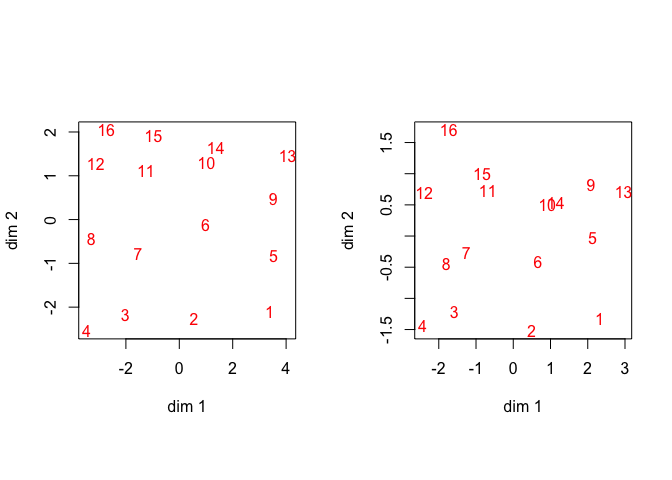Figure 1: Rectangle Data, Configuration, Original Left, Rounded Right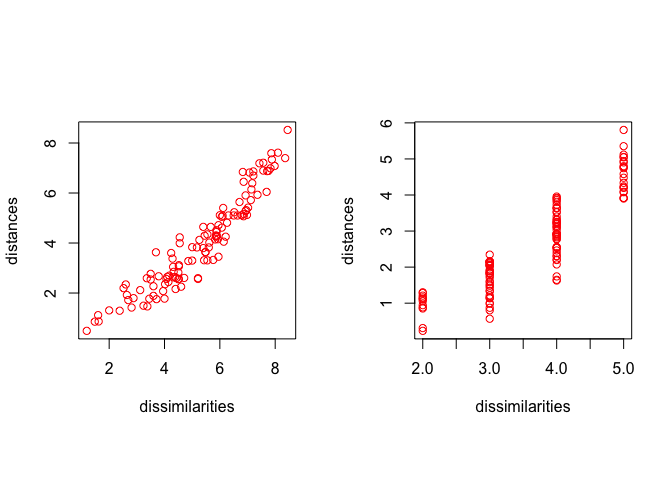Figure 2: Rectangle Data, Shepard Plot, Original Left, Rounded Right

We also compute the solution using loss function $$\eqref{E:KC}$$ with $$\alpha=0$$ instead of $$\eqref{E:final}$$. After 27 iterations we find loss 0.2599461575. The gradient at the solution is

##   +0.048130 +0.028393 -0.001368 +0.037530 +0.041248 -0.061937 +0.018724
##   -0.032240 +0.011883 +0.046100 -0.056752 +0.082335 -0.081614 -0.010317
##  -0.027965 -0.057459 -0.063267 +0.011140 -0.029075 -0.025329 -0.040619
##  +0.028616 +0.009375 -0.049583 -0.015640 -0.046522 +0.034977 -0.001468
##  -0.022992 +0.024503
The solution in figure 3 is virtually indistinguishable from the one in figures 1 and 2.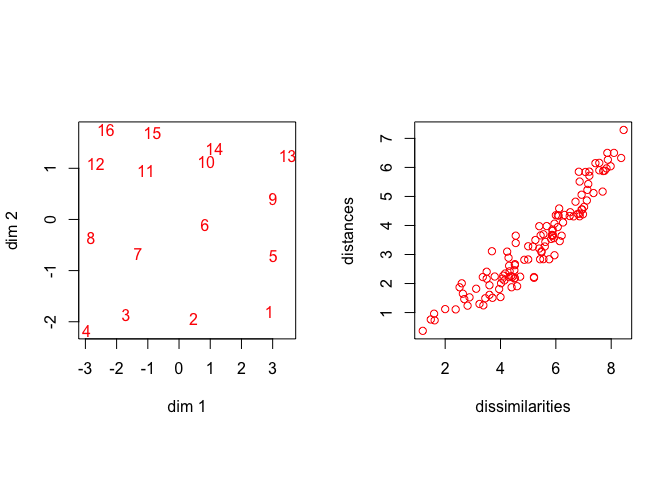Figure 3: Rectangle Data, Normalized by RMS

## 5.2 Dutch Political Parties 1967

As the next illustration we use data from De Gruijter (1967), with average dissimilarity judgments between Dutch political parties in 1967. The data are

##       KVP PvdA  VVD  ARP  CHU  CPN  PSP   BP
## PvdA 5.63
## VVD  5.27 6.72
## ARP  4.60 5.64 5.46
## CHU  4.80 6.22 4.97 3.20
## CPN  7.54 5.12 8.13 7.84 7.80
## PSP  6.73 4.59 7.55 6.73 7.08 4.08
## BP   7.18 7.22 6.90 7.28 6.96 6.34 6.88
## D66  6.17 5.47 4.67 6.13 6.04 7.42 6.36 7.36

The process converges in 1 iterations to loss function value 0.0608337153. The gradient at the solution is

##   -0.046432 +0.026465 -0.050854 +0.039836 -0.017988 -0.003396 -0.062178
##   +0.022516 -0.018144 +0.043513 -0.019920 -0.006859 +0.030020 +0.005290
##  +0.074522 +0.007835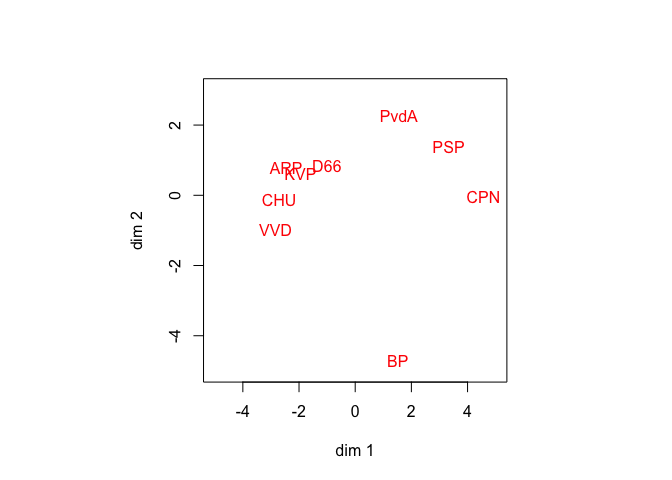Figure 4: De Gruijter Data, Configuration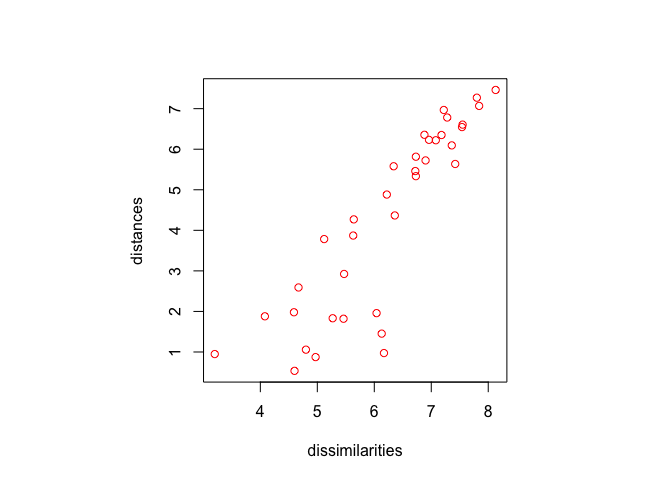Figure 5: De Gruijter Data, Shepard Plot

## 5.3 Ekman Color Data

The final example are the color data from Ekman (1954).

The process converges in 10 iterations to loss function value 0.000691019569788605. The gradient at the solution is

##   -0.000003 -0.000083 +0.000067 -0.000008 +0.000001 -0.000070 -0.000139
##   +0.000072 +0.000017 -0.000003 +0.000032 -0.000007 +0.000078 -0.000037
##  +0.000031 +0.000082 +0.000073 +0.000016 +0.000042 +0.000033 -0.000046
##  +0.000046 -0.000064 +0.000083 +0.000020 +0.000065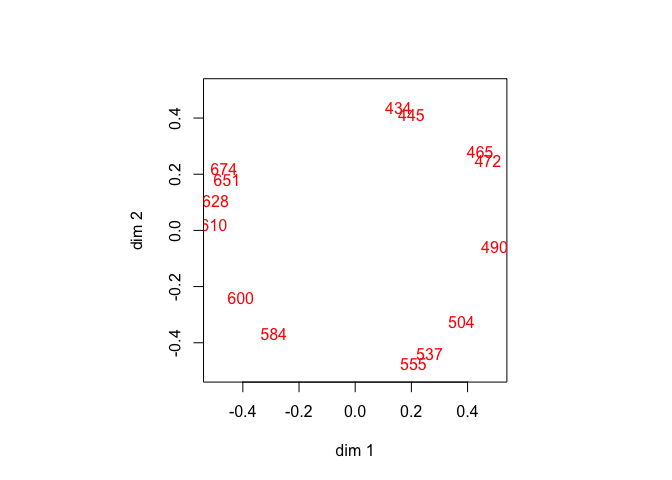Figure 6: Ekman Data, Configuration